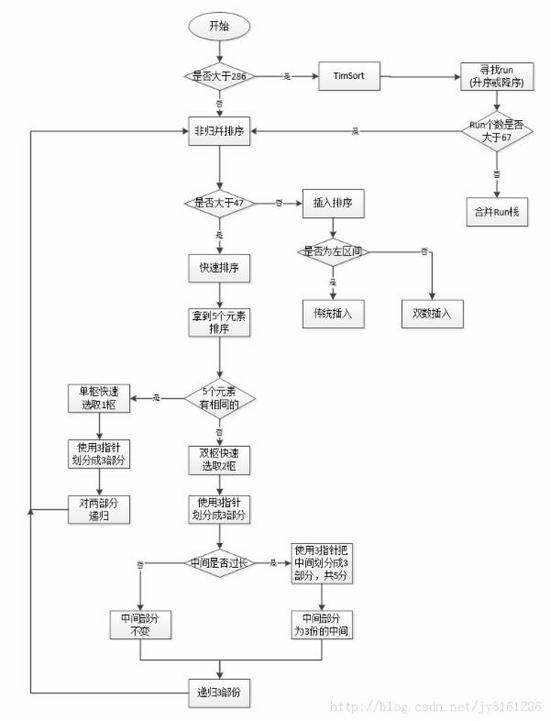# java 快排的思路与算法

2016/03/07 23:19

java 快排的思路与算法

有时候面试的时候的会问道Arrays.sort()是怎么实现的，我以前根本不知道是什么东西，最近点进去看了一下。直接吓傻，

``````  //看到这个时候还是比较淡定的，可怕的事情来了。
public static void sort(int[] a) {
DualPivotQuicksort.sort(a, 0, a.length - 1, null, 0, 0);
}``````

点进这里面看了一下，顿时没有看下去的欲望了。

``DualPivotQuicksort//共有3079行``

从网上找一个分析流程图（算法看流程图是最好的）：图片网址来自http://www.tuicool.com/articles/BfY7Nz（感谢）我们肯定没有办法一下子就写出这样的代码，所以先来了解每个算法的实现是最基本的，后面争取自己整合一个。

这次先实现的快速排序算法（DualPivotQuicksort类名一半是）：

``````public class Sort {
static boolean less(int a, int b){
return a<b;
}

static void exch(int array[],int i,int j){
int temp=array[i];
array[i]=array[j];
array[j]=temp;
}

static void comExch(int array[],int i,int j){
if(less(array[j],array[i]))
exch(array,i,j);
}

//扫描
static int partition(int a[],int l,int r){
int i=l-1,j=r;
int v=a[r];
for(;;){
while(less(a[++i],v));
while(less(v,a[--j])){
if(j==l)break;
}
if(i>=j)break;
exch(a,i,j);
}
exch(a,i,r);
return i;
}
//快速排序
static void quicksort(int a[],int l,int r){
if(r<=l)return;
int i=partition(a, l, r);
quicksort(a,l,i-1);
quicksort(a,i+1,r);
}
public static void main(String args[]){
int array[]={1,3,2,5,4,9,8,0};
quicksort(array,0,7);
for(Integer n:array){
System.out.println(n);
}
}
}``````

设要排序的数组是A……A[N-1]，首先任意选取一个数据（通常选用数组的最后一个数）作为关键数据，然后将所有比它小的数都放到它前面，所有比它大的数都放到它后面，这个过程称为一趟快速排序。值得注意的是，快速排序不是一种稳定的排序算法，也就是说，多个相同的值的相对位置也许会在算法结束时产生变动。

这个只是最简单的快排，只是类的一半，另一半等我搞懂为什么要分轴区的时候再补充算法。

1
7 收藏

### 作者的其它热门文章0 评论
7 收藏
1